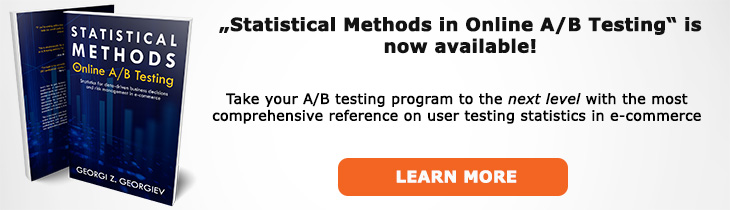# What does "Average Sample Size" mean?

Definition of Average Sample Size in the context of A/B testing (online controlled experiments).

## What is Average Sample Size?

Average sample size is an estimate of the expected sample size in sequential testing where one can perform optional stopping and maintain error guarantees. Unlike a fixed-sample test, a sequential test doesn't have predetermined sample size: depending on the true effect size the test might stop at any of the interim analyses, planned or unplanned (if using an error-spending function).

The average sample is calculated for a point hypothesis alternative by taking the average of all stopping times across an infinite number of hypothetical experiments. It can be calculated for the alternative hypothesis for which the test was powered or any other point from the parameter space. One can also calculate an average of the average with a simple method being to take a uniform distribution over a range of possible values, estimate the average sample size of each and then calculate its arithmetic mean. A non-uniform distribution can be used as well if there is a reliable prognosis about the possible values.

The average sample size is computed as part of an AGILE A/B test.

## Related A/B Testing terms

Error-Spending Function

Sample Space

Alpha-Spending

Beta-Spending

Like this glossary entry? For an in-depth and comprehensive reading on A/B testing stats, check out the book "Statistical Methods in Online A/B Testing" by the author of this glossary, Georgi Georgiev.## Glossary Index by Letter

Select a letter to see all A/B testing terms starting with that letter or visit the Glossary homepage to see all.